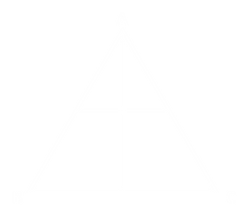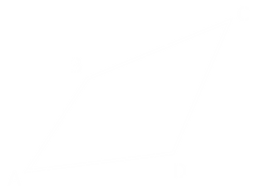top of page
##### Skewness Calculation for 2D Elements

Skewness Calculation:

• Skewness is the Angular Measure of Element quality with respect to the Angles of Ideal Element Types.

• It is one of the Primary Qualities Measures of FE Mesh. Skewness determines how close to ideal (i.e., equilateral or equi-angular) a face or cell is.

• There are two different methods for calculating Skewness for 2D elements.

Method-1: Calculation of Skewness for Triangular/Quadrilateral Elements (Angular Measure)

Triangular Element:

• Draw a line from each node to mid point of its opposite side, Draw another line joining mid-points of other two sides measure the angles between two lines. Repeat the step for all the three nodes and find all six angles (Θ1 to Θ6).

• Skewness is calculated by subtracting Minimum angle from 90 degree.Skewness of an Equilateral Triangle:

For Equilateral Triangle, Θ1, Θ2….. Θ6 = 90 degree

Hence, Skewness = 0 degree

Skewness= 90-min(Θ1, Θ2….. Θ6)• Draw the lines joining the mid points of opposite sides and Measure the Angle between these two lines (Θ1 & Θ2).

• Skewness is calculated by Subtracting the Minimum angles of Θ1 & Θ2 from 90 degrees.Skewness for Square Shaped Element:

• For Square Θ1 & Θ2 = 90 degree

• Hence, Skewness for Square = 0 degree

Skewness = 90-min(Θ1 , Θ2)Note: The acceptable Range of skewness is " 0 ̊ to 45 ̊ " beyond which results may to be close to the actual solution.

Method-2: Calculation of Skewness for Triangular/Quadrilateral Elements (Normalized angle deviation )

In the normalized angle deviation method, skewness is defined (in general) as "maximum of ratio of Angular deviation from Ideal element.θmax = Largest Angle in the face or cell

θmin = Smallest Angle in the face or cell

θe = Angle for an equi-angular face or cell

where, θe = 60 ̊ for Equilateral Triangle and 90 ̊  for Squareθmax = 110 ̊, θmin =30 ̊, θe = 60 ̊###### Skewness for Tria= max(0.42,0.50)= 0.50θmax = 145 ̊, θmin =44 ̊, θe = 90 ̊

###### Skewness for Quad= max(0.61,0.51)= 0.61

Note: The acceptable Range of skewness is "0 to 0.5" beyond which results may to be close to the actual solution.

References:

1. Finite Element Analysis For Design Engineers by Paul M. Kurowski (Chapter 5.3.1).

2. Practical Finite Element Analysis by Nitin S Gokhale, Sanjay S Deshpande, Sanjeev V Bedekar and Anand N Thite (Chapter 7.9).

3. The Finite Element Method : Practical Course by G. R. Liu , S. S. Quek (Chapter 11.4.2).

4. Ansys theory reference manual.

5. Hypermesh users guide.

Note (**): Ansys, Hypermesh are Registered trademarks of their respective owners.

bottom of page